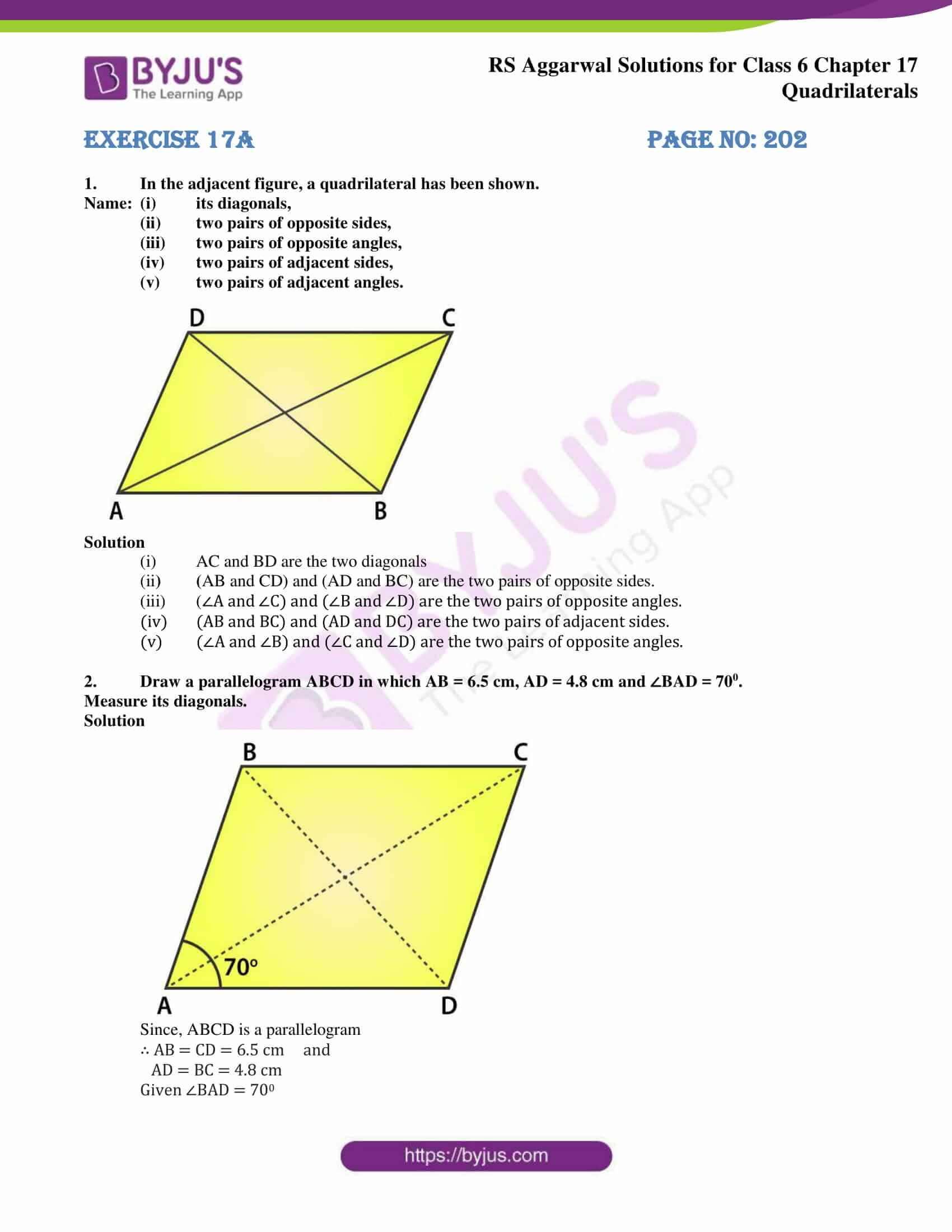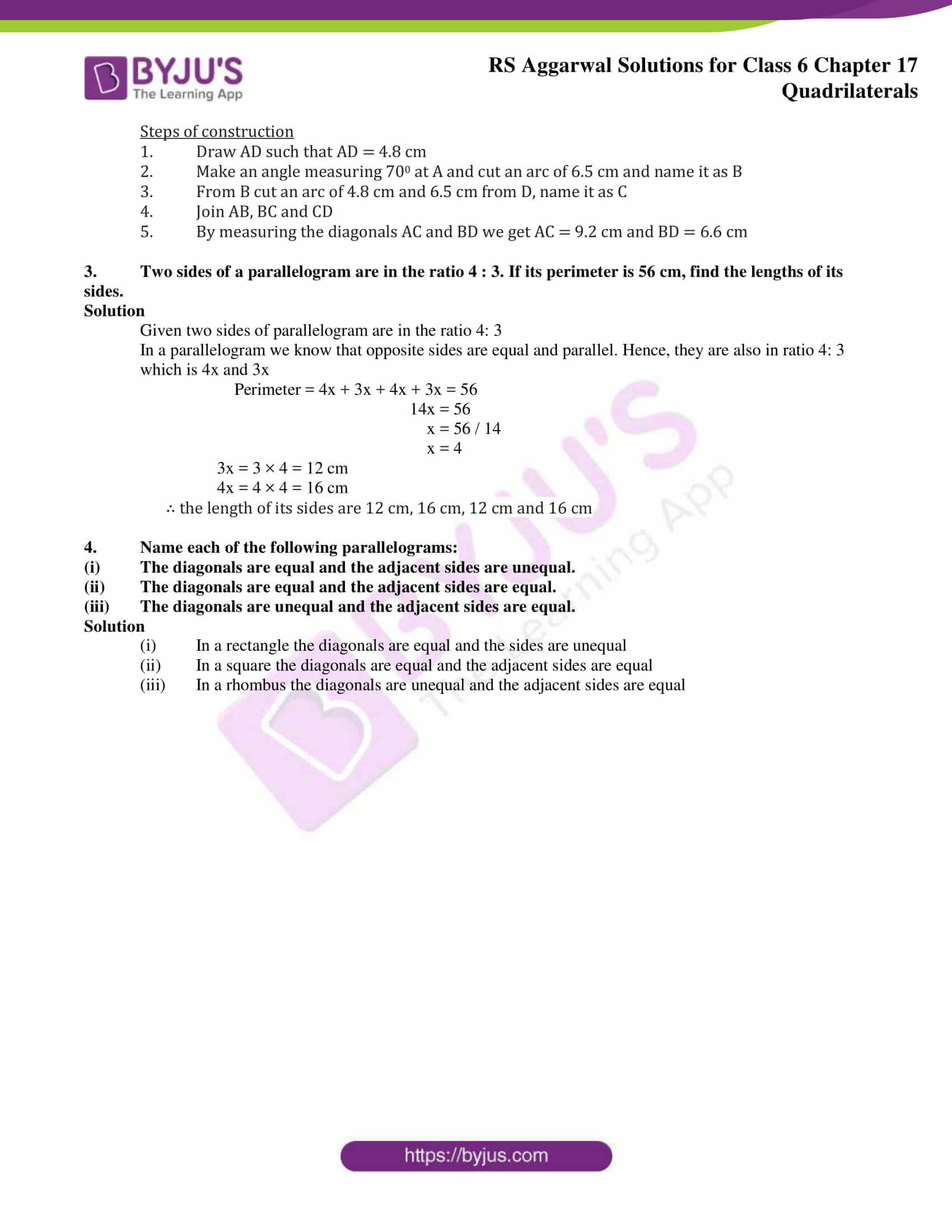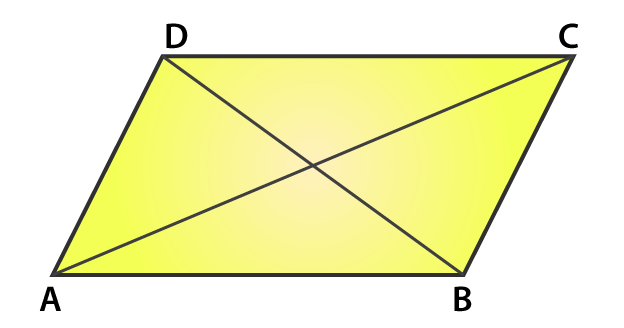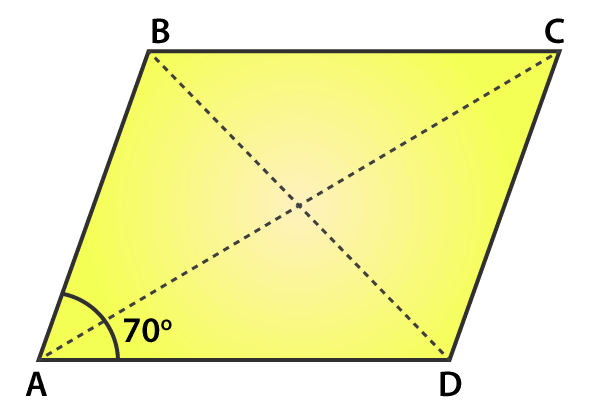# RS Aggarwal Solutions for Class 6 Chapter 17 Quadrilaterals Exercise 17A

In this chapter, students mainly get the basic idea about the quadrilaterals and their types. A simple closed figure which is bound by four line segments is called a quadrilateral. Exercise 17A of RS Aggarwal Solutions has step by step answers which can be grasped easily by the students. The solutions can be downloaded and referred while solving exercise wise problems of RS Aggarwal textbook. RS Aggarwal Solutions for Class 6 Chapter 17 Quadrilaterals Exercise 17A PDF are provided here.### Access answers to Maths RS Aggarwal Solutions for Class 6 Chapter 17 Quadrilaterals Exercise 17A

Name: (i) its diagonals,

(ii) two pairs of opposite sides,

(iii) two pairs of opposite angles,

(iv) two pairs of adjacent sides,

(v) two pairs of adjacent angles.Solution

(i) AC and BD are the two diagonals

(ii) (AB and CD) and (AD and BC) are the two pairs of opposite sides.

(iii) (∠A and ∠C) and (∠B and ∠D) are the two pairs of opposite angles.

(iv) (AB and BC) and (AD and DC) are the two pairs of adjacent sides.

(v) (∠A and ∠B) and (∠C and ∠D) are the two pairs of opposite angles.

2. Draw a parallelogram ABCD in which AB = 6.5 cm, AD = 4.8 cm and ∠BAD = 700.

Measure its diagonals.

SolutionSince, ABCD is a parallelogram

∴ AB = CD = 6.5 cm and

AD = BC = 4.8 cm

Steps of construction

2. Make an angle measuring 700 at A and cut an arc of 6.5 cm and name it as B

3. From B cut an arc of 4.8 cm and 6.5 cm from D, name it as C

4. Join AB, BC and CD

5. By measuring the diagonals AC and BD we get AC = 9.2 cm and BD = 6.6 cm

3. Two sides of a parallelogram are in the ratio 4 : 3. If its perimeter is 56 cm, find the lengths of its sides.

Solution

Given two sides of parallelogram are in the ratio 4: 3

In a parallelogram we know that opposite sides are equal and parallel. Hence, they are also in ratio 4: 3 which is 4x and 3x

Perimeter = 4x + 3x + 4x + 3x = 56

14x = 56

x = 56 / 14

x = 4

3x = 3 × 4 = 12 cm

4x = 4 × 4 = 16 cm

∴ the length of its sides are 12 cm, 16 cm, 12 cm and 16 cm

4. Name each of the following parallelograms:

(i) The diagonals are equal and the adjacent sides are unequal.

(ii) The diagonals are equal and the adjacent sides are equal.

(iii) The diagonals are unequal and the adjacent sides are equal.

Solution

(i) In a rectangle the diagonals are equal and the sides are unequal

(ii) In a square the diagonals are equal and the adjacent sides are equal

(iii) In a rhombus the diagonals are unequal and the adjacent sides are equal

### Access other exercises of RS Aggarwal Solutions for Class 6 Chapter 17 Quadrilaterals

Exercise 17B Solutions